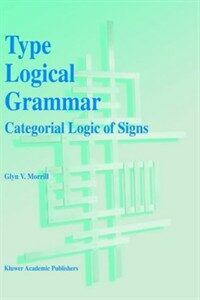> 상세정보

# 상세정보## Type logical grammar : categorial logic of signs (6회 대출)

자료유형
단행본
개인저자
Morrill, Glyn V.
서명 / 저자사항
Type logical grammar : categorial logic of signs / by Glyn V. Morrill.
발행사항
Dordrecht ;   Boston :   Kluwer Academic Publishers,   c1994.
형태사항
xiv, 307 p. : ill. ; 24 cm.
ISBN
0792330951 0792332261 (pbk.)
서지주기
Includes bibliographical references (p. 283-295) and index.
일반주제명
Categorial grammar. Type theory. Semantics (Philosophy). Montague grammar.
비통제주제어
Semantics,,
 000 00000cam u2200205 a 4500 001 000000606077 005 20191011165154 008 940801s1994 ne a b 001 0 eng 010 ▼a 94033169 020 ▼a 0792330951 020 ▼a 0792332261 (pbk.) 040 ▼a DLC ▼c DLC ▼d UKM ▼d 211009 049 1 ▼l 111111420 050 0 0 ▼a P161 ▼b .M67 1994 082 0 0 ▼a 415 ▼2 23 084 ▼a 415 ▼2 DDCK 090 ▼a 415 ▼b M874t 100 1 ▼a Morrill, Glyn V. 245 1 0 ▼a Type logical grammar : ▼b categorial logic of signs / ▼c by Glyn V. Morrill. 260 ▼a Dordrecht ; ▼a Boston : ▼b Kluwer Academic Publishers, ▼c c1994. 300 ▼a xiv, 307 p. : ▼b ill. ; ▼c 24 cm. 504 ▼a Includes bibliographical references (p. 283-295) and index. 650 0 ▼a Categorial grammar. 650 0 ▼a Type theory. 650 0 ▼a Semantics (Philosophy). 650 0 ▼a Montague grammar. 653 0 ▼a Semantics

### 소장정보

No. 소장처 청구기호 등록번호 도서상태 반납예정일 예약 서비스
No. 1 소장처 청구기호 415 M874t 등록번호 111815926 도서상태 대출가능 반납예정일 예약 서비스

### 컨텐츠정보

#### 목차

```
CONTENTS
Notational Conventions = xi
Foreword = xiii
Chapter One: Montague Grammar = 1
1 Model Theoretic Semantics = 4
2 Grammar Terminology and Presentation = 12
3 Montagovian Fragment = 19
3.1 Intensionality = 22
3.2 Object Position = 26
3.3 Quantifying-In = 28
3.4 Coordination and Relativisation = 38
4 Conversion to Direct Semantics = 43
Chapter Two: Logic of Types and Types of Logic = 47
1 The Curry-Howard Correspondence = 48
1.1 Natural Deduction = 51
1.2 Gentzen Sequent Logic = 53
1.3 Conjunction and Disjunction = 55
2 The Structural Hierarchy = 59
Chapter Three: Categorial Grammar = 69
1 Non-Associative Lambek Calculus = 72
2 Associative Lambek Calculus = 78
3 Coordination = 82
Chapter Four: Enriching Categorial Grammar = 87
1 Multimodal Systems = 87
2 Labelled Deduction = 92
3 Discontinuity Operators = 101
3.1 Discontinuous Functors = 113
3.2 Quantifier Raising = 114
3.3 Pied-Piping = 117
3.4 Gapping = 122
3.5 Object-Antecedent Reflexivisation = 127
4 Domain Modalities = 129
Chapter Five: Intensionality and Binding = 137
1 Intensionality = 137
2 Quantification = 149
3 Reflexivisation = 157
Chapter Six: Polymorphism = 161
1 Meet and Join Types = 162
2 Dependent Types = 170
2.1 Dependent Function Types = 171
2.2 Dependent Sum Types = 181
3 Features and Dependent Types = 183
3.1 Nominal Features = 183
3.2 Verbal Features = 187
Chapter Seven: Structural Control = 191
1 Structural Facilitation = 192
1.1 Associativity in NL = 192
1.2 Associativity and Commutativity in NL = 197
1.3 Commutativity in L = 198
1.4 Contraction and Expansion in L = 200
1.5 Contraction and Weakening in L = 201
2 Subalgebra Models = 202
2.1 Groupoid Prosodic Interpretation = 202
2.2 Type Logical Semantic Interpretation = 203
2.3 Subalgebra Model Theory for Structural Facilitation = 204
3 Kripke Models = 207
4 Linguistic Examples = 208
5 Prosodic Phrasing = 213
6 Structural Inhibition = 218
7 Functional and Relational Models for Structural Inhibition = 221
8 Linguistic Examples = 222
Chapter Eight: Extraction = 225
1 Fronting = 226
2 Relativisation = 227
2.1 Obligatory Extraction = 233
2.2 That-less Relatives = 234
2.3 Pied-Piping = 235
2.4 Islands: Prosodic Boundaries = 238
2.5 Subject Extraction = 242
2.6 Differential Penetrability of Islands = 245
3 Refinements . = 246
Chapter Nine: Conclusion = 249
1 Language Science  = 249
2 Categorial History = 250
3 Architecture = 252
4 Transformational History = 255
Appendix A: Interpretation of Types = 263
Appendix B: Gentzen Sequent Rules = 269
Appendix C: Summary Grammar = 277
Bibliography = 283
Index = 297

```